# Forced vibrations without damping

In the introductory tutorial in this series we considered a harmonic forcing function F0cos(ωt) applied to a translational spring and mass system with one degree of freedom having mass m and spring constant k  shown in Figure 1 below with the spring extended by distance x .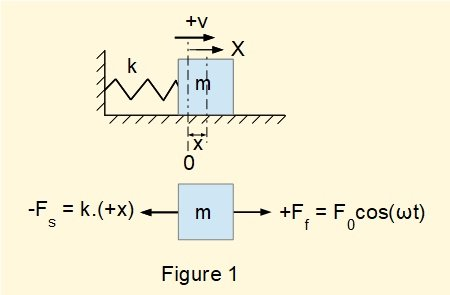It should be understood that a completely undamped spring and mass oscillating system does not exist.  Coulomb friction forces will in time dissipate the initial transient  response after which the forcing function produces a steady state response.   However, analysing the response to a forcing function of a system with no explicit damping term has merit in that it introduces important concepts, particularly resonance

From the dynamic* free body diagram we obtained the following equation of motion in terms of displacement x and time t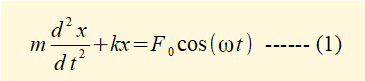*  showing forces producing acceleration of mass m, not static equilibrium

We now find a function x(t) that is a general solution to this equation.  If you are not familiar with solving second-order non-homogeneous ordinary linear differential equations using the method of undetermined coefficients refer to the  maths tutorial.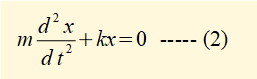From a previous tutorial we know the general solution xh(t) to this equation is: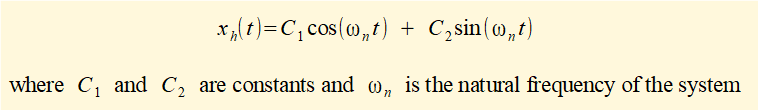We now propose a particular solution xp(t) to equation (1) of the form xp(t) = [A.cos(ωt) + B.sin(ωt)]  where A and B are undetermined coefficients and ω is the frequency of the harmonic forcing function.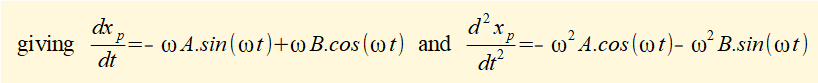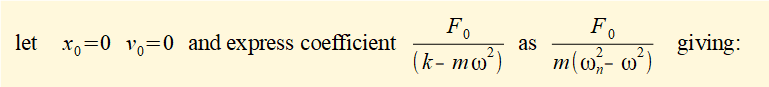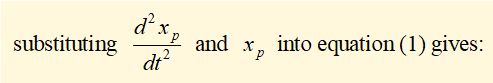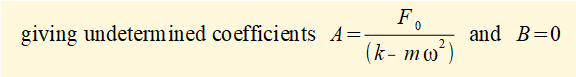The general solution to equation (1) is   xh(t) +xp(t)   giving: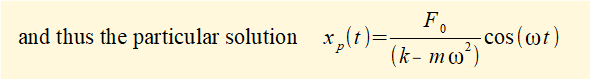Equation (3) is a valid solution for all values of ω except ω = ωn  which gives a duplicate solution containing the function cos(ωnt).  The proposed particular solution for this condition takes the form xp(t) = [A.t.cos(ωnt) + B.t.sin(ωnt)] which we develop later.  Meanwhile continuing with a particular solution to equation (1) for ω ≠ ωn we find constants C1 and C2 for initial conditions x0 and v0  at time t = 0 where v0 = dx(t)/dt.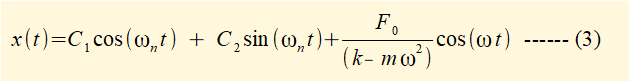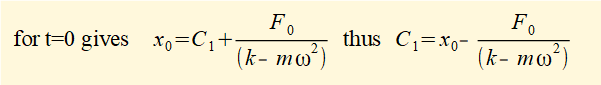Thus the particular solution for equation (1) for initial conditions x0 and v0 is: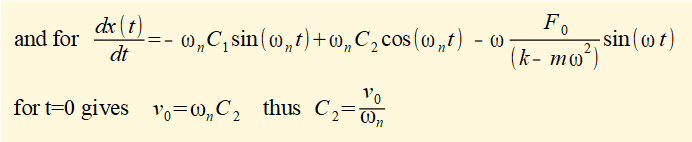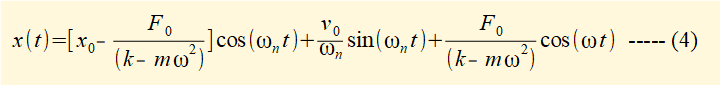The first term in equation (5) with cos(ωnt) expresses the transient response attributable to the free vibration.  In practice this response dissipates because of friction.  The second term with cos(ωt) derived from the particular solution xp(t) expresses the steady state response.

Characteristics of the steady state response x(t) are illustrated in Figures 2 - 5 below.

A useful parameter is the static amplitude δ = (F0 / k) of the forcing function expressing the static displacement of mass m under force F0. Hence the factor (X / δ) is a measure of the amplification effected by the forcing function where X is the amplitude of the harmonic steady state response x(t).Figure 2 plots the steady state response of the system when the angular frequency of the forcing function ω > ωnn is the natural frequency of the system): note the response is out of phase with the forcing function by 180°.  In this example ω = 1.26. ωn   and the amplification factor (X / δ)  ≈ 1.6.Figure 3 plots the steady state response of the system when the angular frequency of the forcing function ω < ωn: note the response is in phase with the forcing function.  In this example ω = 0.73. ωn   and the amplification factor (X / δ)  ≈ 2.2.

The characteristics illustrated in Figures 2 and 3 can be deduced from the relative values of ω and ωn and the sign of (ωn2 - ω2) in the coefficient of the cos(ωt) term in equation (5).  Figure 4 below shows amplification factor (X / δ)  ≈ 10 when ω = 0.95 ωn .Figure 5 below shows the relationship between the frequency ratio (ω/ωn) and the absolute value of amplification factor (X / δ) .  Note: (i) different characteristics for ω<ωn and ω>ωn, (ii) an exponential relationship as ω approaches the value of ωn from either direction.  This condition is known as resonance where the theoretical response tends to infinity.  In practice physical constraints limit the amplitude of vibration.Figures 2-5 illustrate the steady state condition of a free vibrating system subjected to a harmonic forcing function after transient effects are eliminated by the small amount of damping inherent in the system.  We now consider the response including the transient term cos(ωnt) in equation (5).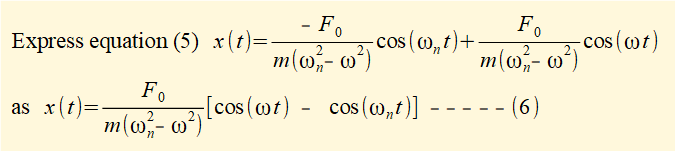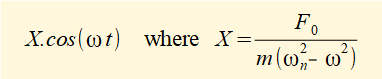Equation (7) is plotted in Figure 6 below for  ω = 40 rad/s and ωn = 30 rad/s giving: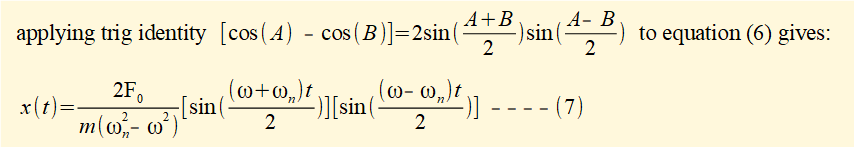This response illustrates the phenomenon of beating where the amplitude of displacement varies periodically  In this example the displacement is a function of sin(35t) and the envelope of the beat is a function of sin(5t).  Beating occurs as an interference phenomenon in sound waves.

The final stage of this analysis is to find the separate solution to equation (3) for ω = ωn .  As noted above the proposed particular solution for this condition takes the form*  xp(t) = [A.t.cos(ωnt) + B.t.sin(ωnt)] ------- (9)

* by the reduction of order method - see the maths tutorial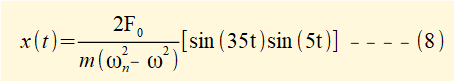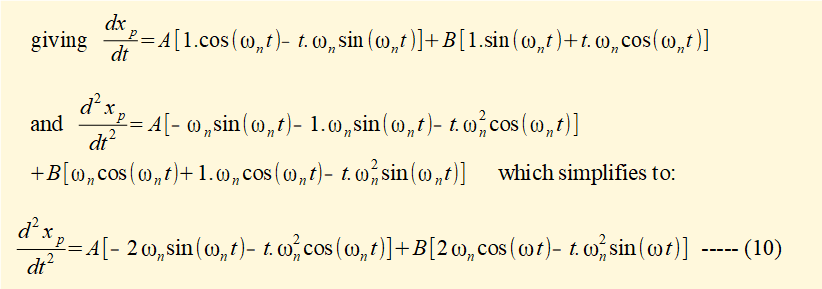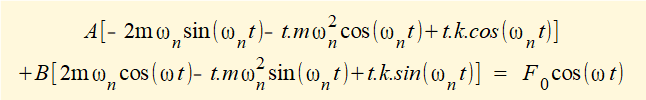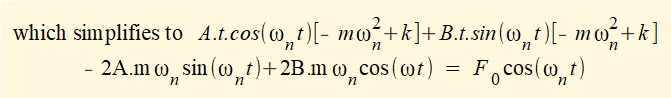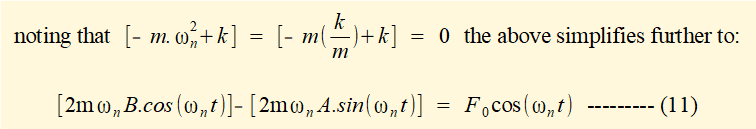The general solution to equation (1) for ω = ωn is   xh(t) +xp(t)   giving: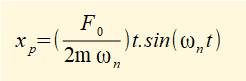From equation (11) find constants C1 and C2 for initial conditions x0 and v0  at time t = 0 where v0 = dx(t)/dt

gives x0 = C1 + 0 + 0    thus C1 = x0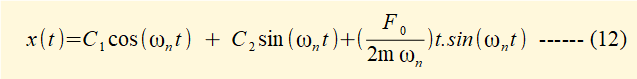Thus the particular solution for equation (1)  when ω = ωn with initial conditions x0 and v0 is: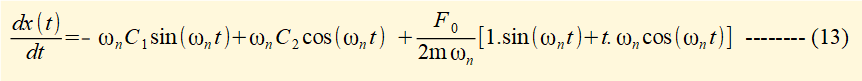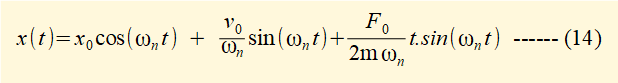Figure 7 below plots x(t) as stated in equation (13).This plot shows the resonant condition induced by forcing function F0cos(ωt) when ω = ωn  implied by the response for ω ≠ ωn  illustrated in Figure 5.  Note that line x(t) = (F0 / 2mωn).t expresses the increase in amplitude of the vibration. Note also the harmonic response (sin(ωnt)) and forcing function (cos(ωnt)) are out of phase by 90°.

The next tutorial examines the response of damped free vibrations to a harmonic forcing function taking account of a damping term in the equation of motion.

I welcome feedback at:

### Tutorials - mechanical vibrations

###### Forced vibrations with damping

Alistair's tutorials 2021